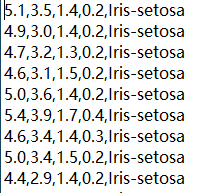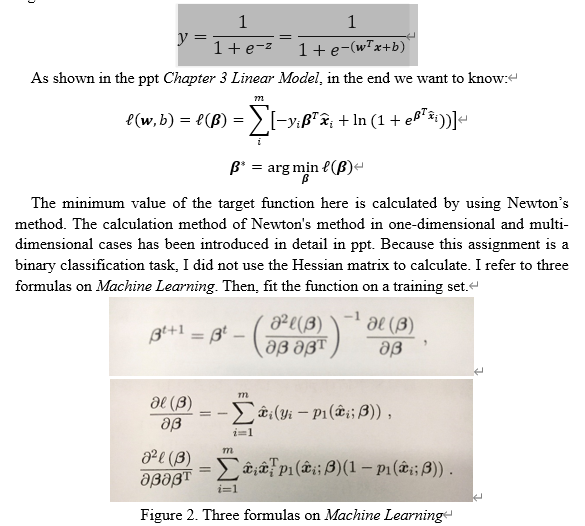# 【模式识别小作业】逻辑回归模型（logistic regression model）+Matlab实现+UCI的Iris和Seeds数据集+分类问题

## 1.Inroduction

In this assignment, I implemented the logistic regression model based on two data sets by using MATLAB. The data sets, Iris and Seeds, are downloaded from the UCI Machine Learning Repository.

Each program contains two .m file. One is to estimate the values of w and b based on the data set and calculate the results on the test data set. The other is to select two attributes from the data set, calculate again, and plot a figure.

Experimental results show that using the logistic regression model on Iris data set to solve the two-classification problems looks good. Because, I think, the number of the sample and attribute of Iris is little, the processing speed is fast and accuracy is high. After matching w and b on the train data set, the samples on the test data set can be classified correctly by this function. While the effect on Seeds data set is not good. Due to the high dimension, many samples and the need of iteration, the speed processing on my computer is so slow that I cannot debug it well.

## 2.The characteristics of the data sets

2.1 Iris
The data set contains 150 samples. Every sample have 4 attributes: sepal length in cm, sepal width in cm, petal length in cm, and petal width in cm. Three classes of Iris is Iris Setosa, Iris Versicolour and Iris Virginica. This data set is the most popular in UCI. The clear structure and plentiful samples make it suitable in this assignment.

2.2 Seeds
The data set contains 210 samples. Every sample have 7 attributes: area A, perimeter P, compactness C = 4piA/P^2, length of kernel, width of kernel, asymmetry coefficient and length of kernel groove. And all of these parameters were real-valued continuous. The data set comprises kernels belonging to three different varieties of wheat: Kama, Rosa and Canadian. It is often used for the tasks of classification and cluster analysis.

## 3.Data preprocessing

Taking the Iris as an example, the first step is to convert characters in data set into digital representations.Read the dataset file with MATLAB, extract the whole attributes into an array, and merge the numeric symbols after each sample. In order to practice the two-category problem, the first two flowers are extracted from the original data set. Iris-setosa is marked as 0, and Iris-versicolor is marked as 1. Then, the hold-out method is used to extract one out of every 5 samples to form a test set. The remaining 80 samples are used as the training set. And write these data set to each txt file to facilitate saving and using.

## 4.Design of the modules

In this assignment, the Newton’s method is used to compute the optimal solution of w and b. Firstly, set set the initial value of β, which is the merge of w and b. The binary classification task with labels from {0, 1} is considered: 𝑧=𝒘^𝑇 𝒙+𝑏∈ℝ→ {0, 1}. The logistic function is:The code:

%This program is based on the data set Iris
%The original data set contains three kinds of flowers
%In order to practice the two-category problem
%The first two flowers are extracted from the original data set
%And the flower name in the data set is changed to a numeric symbol of 0, 1

clear;
clc;

%%%%%%%%%%%%%%Data preprocessing section%%%%%%%%%%%%%%%%
f=fopen('iris.data');%Open dataset file
data=textscan(f,'%f,%f,%f,%f,%s'); %Read file content

D=[];% Used to store attribute values
for i=1:length(data)-1
D=[D data{1,i}];
end
fclose(f);

lable=data{1,length(data)};
n1=0;n2=0;n3=0;
% Find the index of each type of data
for j=1:length(lable)
if strcmp(lable{j,1},'Iris-setosa')
n1=n1+1;
index_1(n1)=j;% Record the index belonging to the "Iris-setosa" class

elseif strcmp(lable{j,1},'Iris-versicolor')
n2=n2+1;
index_2(n2)=j;

%    elseif strcmp(lable{j,1},'Iris-virginica')
%        n3=n3+1;
%        index_3(n3)=j;

end
end

% Retrieve each type of data according to the index
class_1=D(index_1,:);
class_2=D(index_2,:);
% class_3=D(index_3,:);
Attributes=[class_1;class_2];%class_3];

I=[0*ones(n1,1);1*ones(n2,1)];%2*ones(n3,1)]; %Iris-setosa is marked as 0; Iris-versicolor is marked as 1
Iris=[Attributes I];% Change the name of the flower to a number tag

save Iris.mat Iris % Save all extracted data as a mat file

%Save all extracted data as a txt file
f=fopen('iris1.txt','w');
[m,n]=size(Iris);
for i=1:m
for j=1:n
if j==n
fprintf(f,'%g \n',Iris(i,j));
else
fprintf(f,'%g,',Iris(i,j));
end
end
end
fclose(f);

%Use the set-out method to extract one out of every 5 data, a total of 20 data to form a test set
f_test=fopen('iris_test.txt','w');
[m,n]=size(Iris);
for i=1:m
if rem(i,5)==0
for j=1:n
if j==n
fprintf(f_test,'%g \n',Iris(i,j));
else
fprintf(f_test,'%g,',Iris(i,j));
end
end
end
end
fclose(f_test);

%The remaining 80 data as a training set
f_train=fopen('iris_train.txt','w');
[m,n]=size(Iris);
for i=1:m
if rem(i,5) ~=0
for j=1:n
if j==n
fprintf(f_train,'%g \n',Iris(i,j));
else
fprintf(f_train,'%g,',Iris(i,j));
end
end
end
end
fclose(f_train);

%%%%%%%%%%%%%%%%%%%%Estimate w and b %%%%%%%%%%%%%%%%%%

baita=[0.25;0.25;0.25;0.25;0]; %Four properties, set the initial value of baita

lable_iris=iris_data(:,5); %Read the floral label in the fifth column
attributes_iris=iris_data(:,1:4); %Read four attribute values
qiudaoone=0;
qiudaotwo=0;

for flag=1:10000000 % Number of iterations
for a=1:1:80
xi=[attributes_iris(a,1);attributes_iris(a,2);attributes_iris(a,3);attributes_iris(a,4);1];%Xi matrix construction
p0=1/(1+exp(baita'*xi)); %P0 probability calculation
p1=1-p0; %P1 probability calculation
qiudaoone=qiudaoone+(xi*(lable_iris(a,1)-p1)); %Machine learning textbook 3.30
qiudaotwo=qiudaotwo+(xi*xi'*p1*(1-p1)); %Machine learning textbook 3.31
end
qiudaoone=qiudaoone*(-1);
baita=baita-inv(qiudaotwo)*qiudaoone; %Machine learning textbook 3.29

end

attributes_test=iris_testdata(:,1:4); %Read four attribute values

f_baita=fopen('iris_baita.txt','w');
for b=1:1:5
fprintf(f_baita,'%g \n',baita(b)); %Write the baita value to the txt file
end
fclose(f_baita);

f_result=fopen('iris_result.txt','w');
for b=1:1:20 %Calculate the test results of the entire test set
result(b)=baita(1)*attributes_test(b,1)+baita(2)*attributes_test(b,2)+baita(3)*attributes_test(b,3)+baita(4)*attributes_test(b,4)+baita(5);
fprintf(f_result,'%g \n',result(b)); %Write the test results to a txt file
end
fclose(f_result);



## 5.Limitations and improvements

1. This logistic regression model can be applied to the second data set Seeds, but the effect is not good. It deserves improving further.
2. Due to the limitations of the computer configuration, I can't debug the code very smoothly. I hope I can have a better device to debug the program later.
3. The principle and algorithm of Newton's method are not particularly clear for me, and further familiarity are needed.
4. Only binary classification tasks are considered in this assignment, and application of the logistic regression model to the multi-classification tasks should be tried in the future.

### 评论

###### Your browser is out-of-date!

Update your browser to view this website correctly. Update my browser now

×